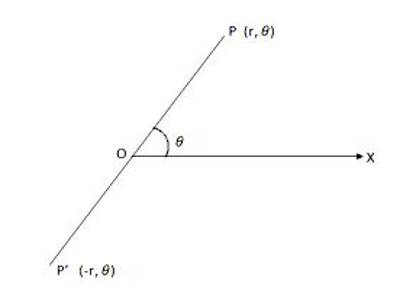Polar Co-ordinates are widely used in higher mathematics as well as in other branches of science. In mathematics, the polar coordinate system is a two-dimensional coordinate system in which each point on a plane is determined by a distance from a reference point and an angle from a reference direction.

In polar co-ordinate system, the position of a point on the reference plane is uniquely determined referred to a fixed point on the plane and a half line drawn through the fixed point. The fixed point is called the Pole or Origin and the half line is drawn through the pole is called the Initial Line.Let OX be the initial line drawn through the pole O on the plane of reference. Take any point P on the plane and join OP.

If OP = r and ∠XOP = θ then the real numbers r and θ are together called the Polar Co-ordinates of P and denoted by (r, θ); here OP. If OP = r and Polar Co-ordinates of P and denoted by (r, θ); here OP = r is called the Radius Vector and ∠XOP = θ, the Vectorial Angle of P. the angle θ is measured by the method of measurement of trigonometrical angle i.e., θ is taken to be positive when it is measured in the anti-clockwise sense from the initial line and negative when it is measured in the clockwise sense from the initial line.

By convection, to represent the polar co-ordinate of a point we first write the radius vector (r) and then the vectorial angle (θ) and they are put together in braces putting a comma between them.

Note:

(i) for given values of r and θ we shall get one and only one point on the reference plane; conversely, for a given point on the plane ‘r’ possesses a definite finite value but θ can have infinite number of value (viz ., θ, 2π + θ, 4π + θ,…….etc.).

(ii) The polar Co-ordinates of the pole are assumed to be (0, 0).

(iii) If the sense of radius vector is taken into account then the value of r may be negative. Thus, if the direction from O to P is taken as positive then the direction from P to O will be negative. Hence, if the points P, O, P’ are collinear such that OP = OP’ = r and ∠XOP = θ then the polar co-ordinates of P and P’ are (r, θ) and (-r, θ) respectively.

However, in practice, it is convenient to take both the radius vector (r) and the vectorial angle (θ) as positive.

(iv) Remembering the rules regarding the signs of r and θ we can represent the polar co-ordinate of P in following different ways:

(r, θ); (-r, π + θ); [r, -(2π – θ)]; [-r, -(π – θ)].

Information Source: